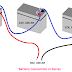Hi, in this article we are going to know the proper battery connection in series and parallel. Also, we will discuss how you can calculate the current and voltage rating according to the type of connection of battery. There are mainly three types of connections are used for the battery with the aim of requirement of capacity. Those three connections are - 1. Series Connection 2. Parallel Connection 3. Series and Parallel Combination. This knowledge helps a lot to understand the capacity of a whole battery bank, or when you going to make a battery bank for UPS or Inverter system.

## Battery Connection in Series

We need to do connect multiple batteries in series when we required more voltage than a single battery voltage. Here you can see three batteries are connected in series.

In the series connection of the batteries, the total voltage will increase while the current capacity remains the same. Here, you can see in the above figure, three 12V, 100AH batteries are connected in series. So the total capacity of the output will be 36V, 100AH. Remember that while connecting batteries in series, all batteries should be in the same category and in good condition. If one of them is damaged or not able to provide the rated voltage, then the output voltage of the circuit also be affected.

## Battery Connection in Parallel

When we required the high ampere-hour capacity, then we need to connect batteries in parallel. Here you can see in the below figure, three batteries are connected in parallel.

Here, you can see three 12V, 100AH batteries are connected in parallel. So the capacity of the output of the circuit will be 12V, 300AH. Here also all batteries should be in good condition. In a series connection, if any one battery is damaged then the total output voltage will be affected but in the parallel connection, if any battery damaged or not able to provide full rated voltage then the output AH capacity of the circuit will be affected.

## Connection of Battery in Series and Parallel Combination

Multiple batteries are needed to connect in series and parallel combination when both output voltage and ampere-hour need as per our requirement.

Here, you can see a total of six batteries are connected in series and parallel combination. Row 1 consists of three batteries(12V, 100AH) connected in series. So the output of Row 1 will be 36V, 100AH.
Row 2 also consists of three batteries(36V, 100AH) connected in series. So the output of Row 2 also is 36V, 100AH.
Now both rows are connected in parallel. So, the output of the total circuit will be 36V, 200AH.

## What happens if we connect batteries with different polarities?

Here we have taken four wrong connections of batteries. Now we will understand what will happen in those four conditions.

In figure A, you can see both are the 12V, 100A batteries and they are connected to each other with opposite polarities in parallel connection. So in this case, you will not get any output or equivalent voltage because they will minimize the voltage to each other.

In figure B, you can see two batteries are connected with opposite polarities in parallel connection but they have different voltage rating 12V and 24V. In this case, you will get only 12V in output for a few seconds only. After that, both batteries will quickly discharge as they create a dead short circuit.

In figure C, you can see two batteries are connected in series. Here, also you cannot get any voltage.

In figure D, you can see two batteries are connected in series but they have different voltage ratings. Here you will get some voltage which will be equal to the 24V with a series resistance of the 12V battery.

Battery Connection Diagram in Series and ParallelReviewed by Author on 5/16/2021 Rating: 5